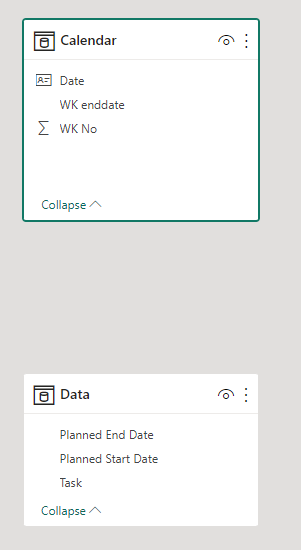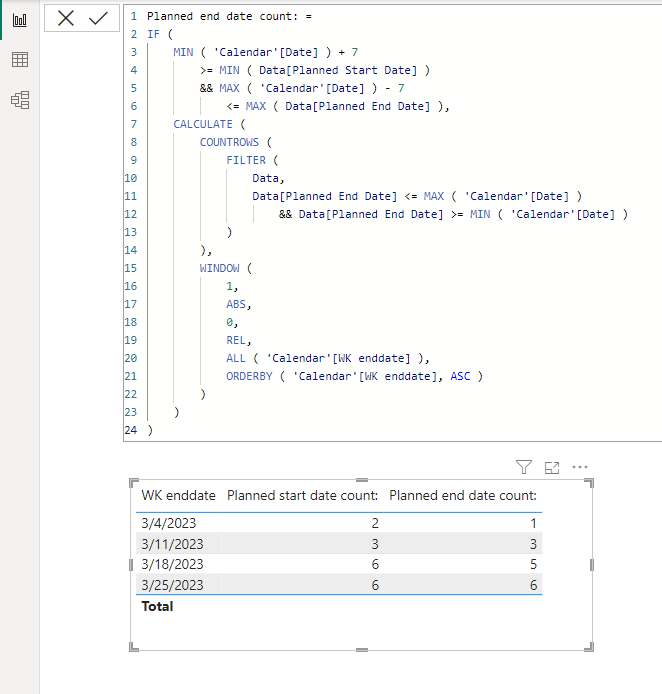cancel
Showing results for
Did you mean:Helper II

## Cumulative data in Dax

Hi,

I have 3 fileds which are Task, Planned Start Date and Planned End Date. I want calculate the cumulative count of Task based on the weekend(All Previous week+ Current week).

Raw Data:

Expected Format:

Note : "Planned Start Date Count Calulation" and "Planned End Date Count Calulation" are just shown for calculation which is not required in visual.

 Week End Date Planned Start Date Count Planned End Date Count Planned Start Date Count Calulation Planned End Date Count Calulation 4-Mar-23 2 1 2 1 11-Mar-23 3 3 2+1 1+2 18-Mar-23 6 5 2+1+3 1+2+2 25-Mar-23 6 6 2+1+3+0 1+2+2+1
1 ACCEPTED SOLUTIONSuper User

Hi,

I am not sure if I understood your question correctly, but please check the below picture and the attached pbix file.``````Planned start date count: =
IF (
MIN ( 'Calendar'[Date] ) + 7
>= MIN ( Data[Planned Start Date] )
&& MAX ( 'Calendar'[Date] ) - 7
<= MAX ( Data[Planned End Date] ),
CALCULATE (
COUNTROWS (
FILTER (
Data,
Data[Planned Start Date] <= MAX ( 'Calendar'[Date] )
&& Data[Planned Start Date] >= MIN ( 'Calendar'[Date] )
)
),
WINDOW (
1,
ABS,
0,
REL,
ALL ( 'Calendar'[WK enddate] ),
ORDERBY ( 'Calendar'[WK enddate], ASC )
)
)
)
``````

``````Planned end date count: =
IF (
MIN ( 'Calendar'[Date] ) + 7
>= MIN ( Data[Planned Start Date] )
&& MAX ( 'Calendar'[Date] ) - 7
<= MAX ( Data[Planned End Date] ),
CALCULATE (
COUNTROWS (
FILTER (
Data,
Data[Planned End Date] <= MAX ( 'Calendar'[Date] )
&& Data[Planned End Date] >= MIN ( 'Calendar'[Date] )
)
),
WINDOW (
1,
ABS,
0,
REL,
ALL ( 'Calendar'[WK enddate] ),
ORDERBY ( 'Calendar'[WK enddate], ASC )
)
)
)``````

If this post helps, then please consider accepting it as the solution to help other members find it faster, and give a big thumbs up.Super User

Hi,

I am not sure if I understood your question correctly, but please check the below picture and the attached pbix file.``````Planned start date count: =
IF (
MIN ( 'Calendar'[Date] ) + 7
>= MIN ( Data[Planned Start Date] )
&& MAX ( 'Calendar'[Date] ) - 7
<= MAX ( Data[Planned End Date] ),
CALCULATE (
COUNTROWS (
FILTER (
Data,
Data[Planned Start Date] <= MAX ( 'Calendar'[Date] )
&& Data[Planned Start Date] >= MIN ( 'Calendar'[Date] )
)
),
WINDOW (
1,
ABS,
0,
REL,
ALL ( 'Calendar'[WK enddate] ),
ORDERBY ( 'Calendar'[WK enddate], ASC )
)
)
)
``````

``````Planned end date count: =
IF (
MIN ( 'Calendar'[Date] ) + 7
>= MIN ( Data[Planned Start Date] )
&& MAX ( 'Calendar'[Date] ) - 7
<= MAX ( Data[Planned End Date] ),
CALCULATE (
COUNTROWS (
FILTER (
Data,
Data[Planned End Date] <= MAX ( 'Calendar'[Date] )
&& Data[Planned End Date] >= MIN ( 'Calendar'[Date] )
)
),
WINDOW (
1,
ABS,
0,
REL,
ALL ( 'Calendar'[WK enddate] ),
ORDERBY ( 'Calendar'[WK enddate], ASC )
)
)
)``````

If this post helps, then please consider accepting it as the solution to help other members find it faster, and give a big thumbs up.